Press the right key for the next slide (or swipe left)

also ...

Press the left key to go backwards (or swipe right)

Press n to toggle whether notes are shown (no equivalent if you don't have a keyboard)

Press m or double tap to see a menu of slides

## ∧Intro and ∨Intro: Compare and Contrast

How come we can prove only valid arguments?

How come we can prove only valid arguments?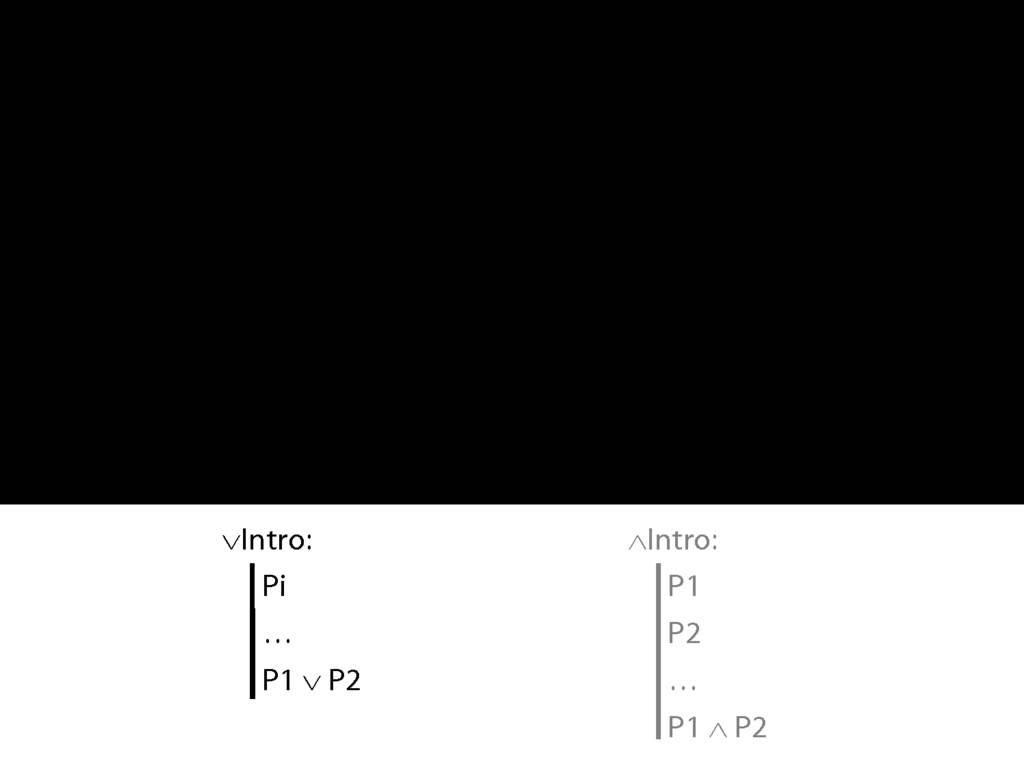Here is a new rule of proof, called disjunction intro.
∨Intro is saying that if you have any sentence, you can add a line to your proof containing a disjunction of that sentence and any other sentence you like.
Explain ∨Intro with an example: if you know Ayesha is in the garden but don’t want to give this away but do want to speak the truth you can say “Ayesha is in the library or Ayesha is in the garden”.
The transition from 'Ayesha is in the garden' to 'Ayesha is in the garden or Ayesha is in the library' is the transition that disjunction intro allows you to make in formal proofs.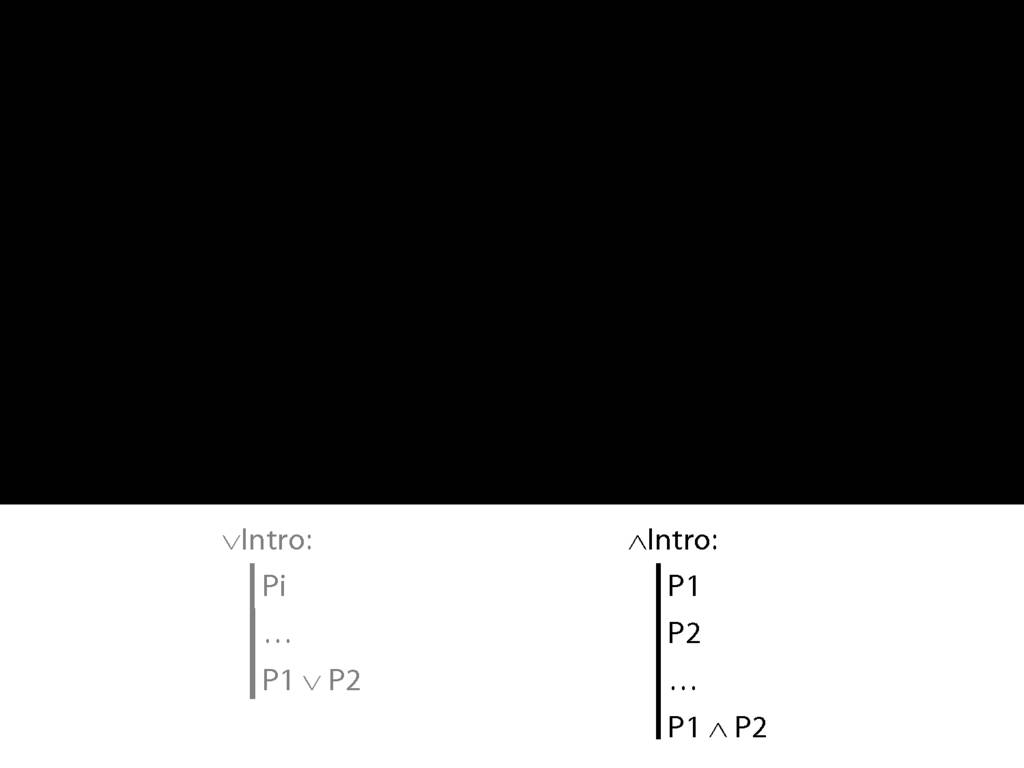Contrast ∧Intro ...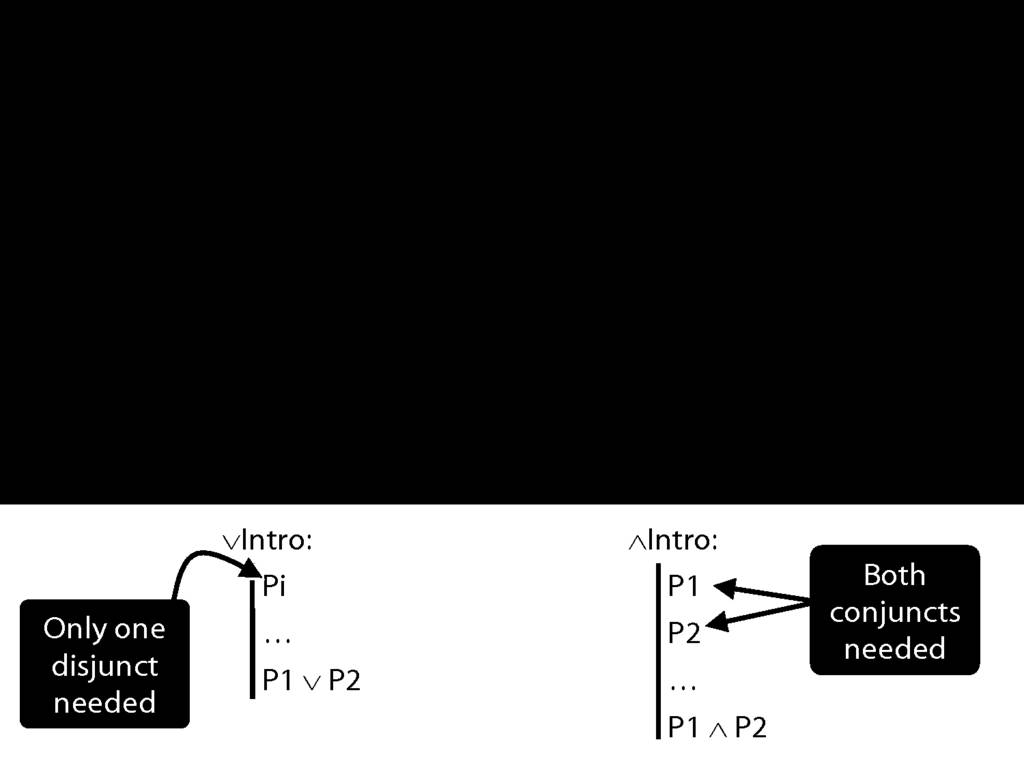∧Intro is just like ∨Intro except that you need both conjuncts for ∧Intro.
Why should we have set things up like this? Why is using ∧Intro more demanding than using ∨Intro?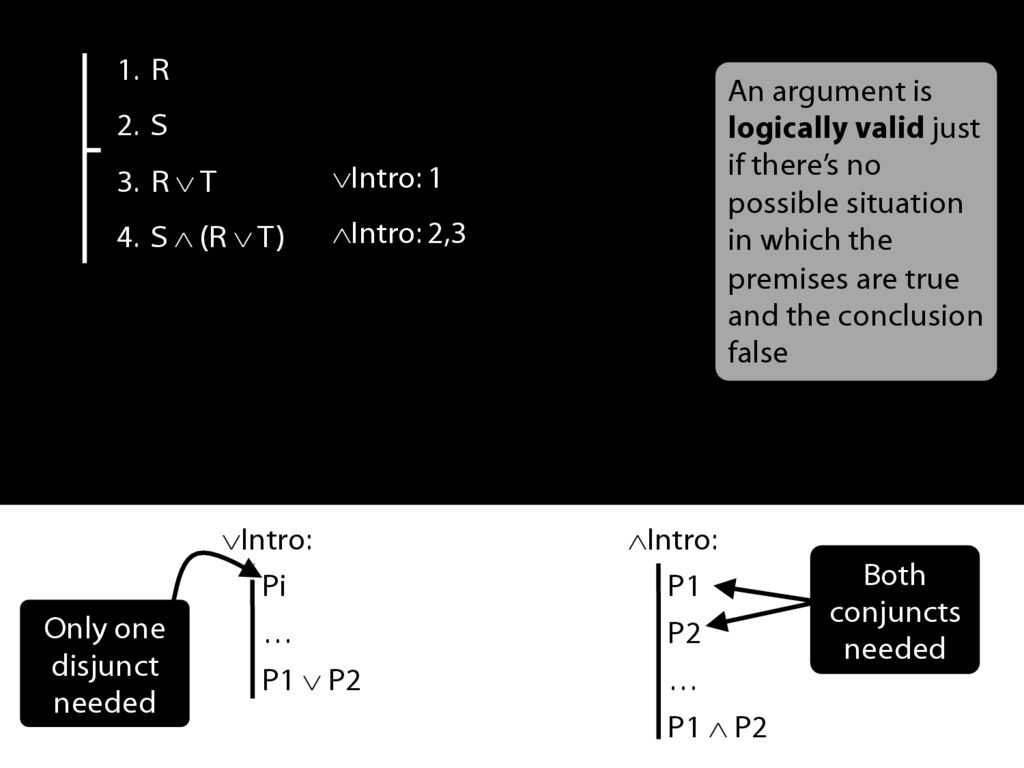Let's have a look at a proof involving these two rules.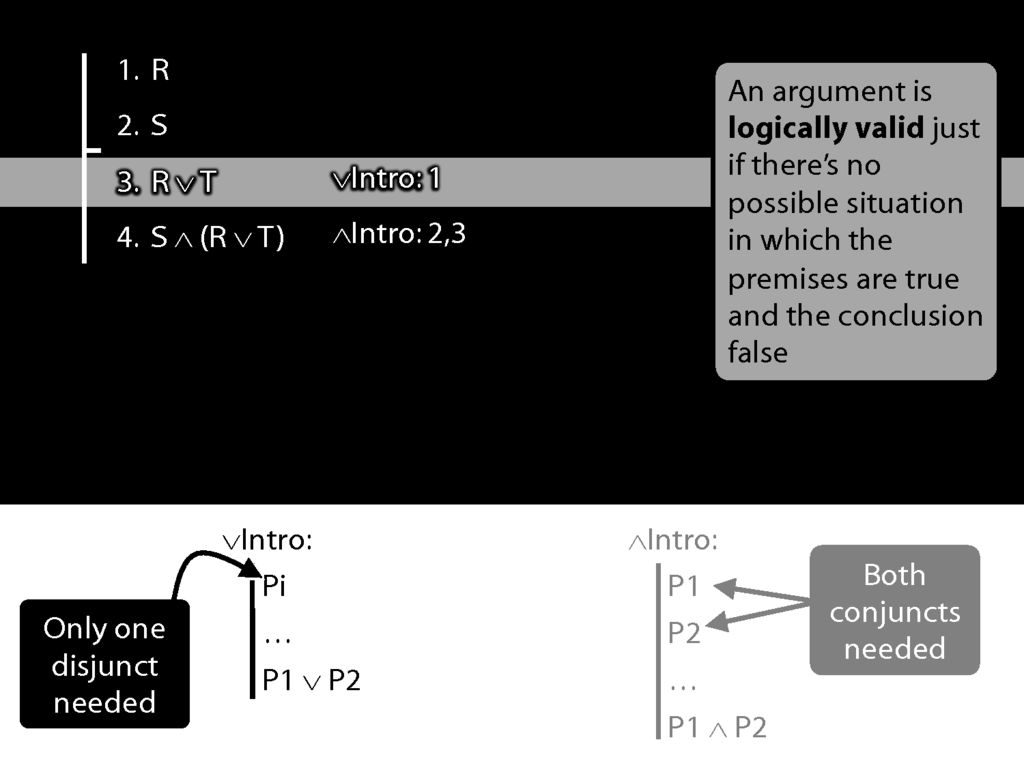We're using ∨Intro at line 3 to get R∨T.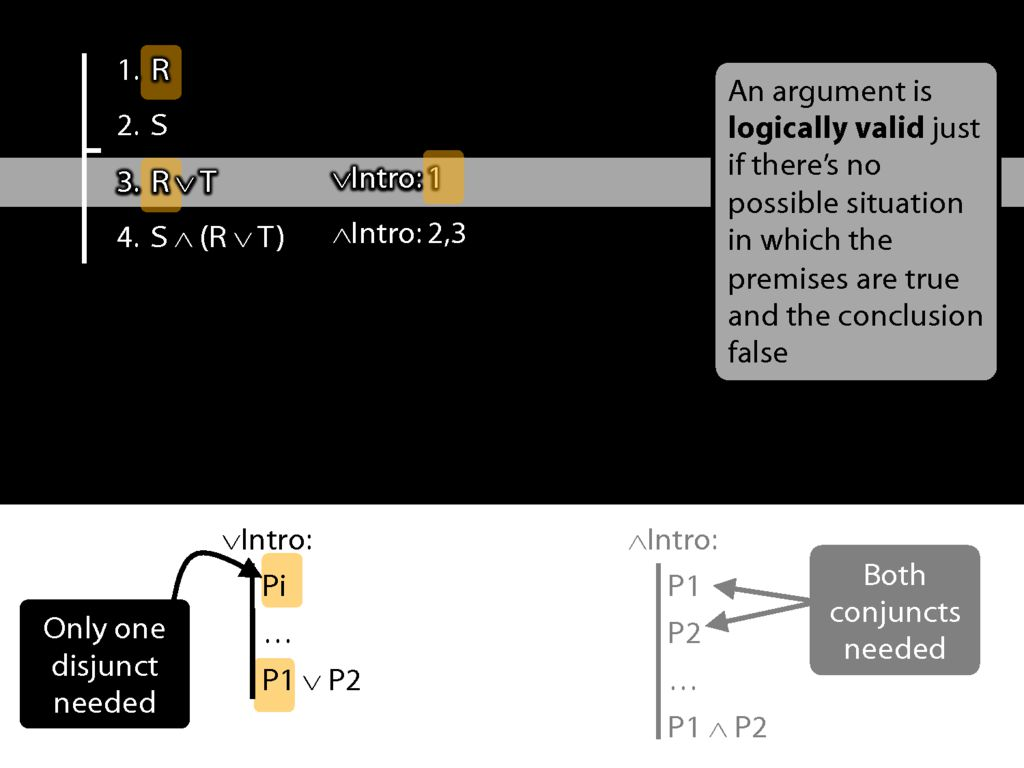See how what we've done matches the rule. (Sometimes people ask, Where does the T come from? The answer is: it just comes from nowhere.)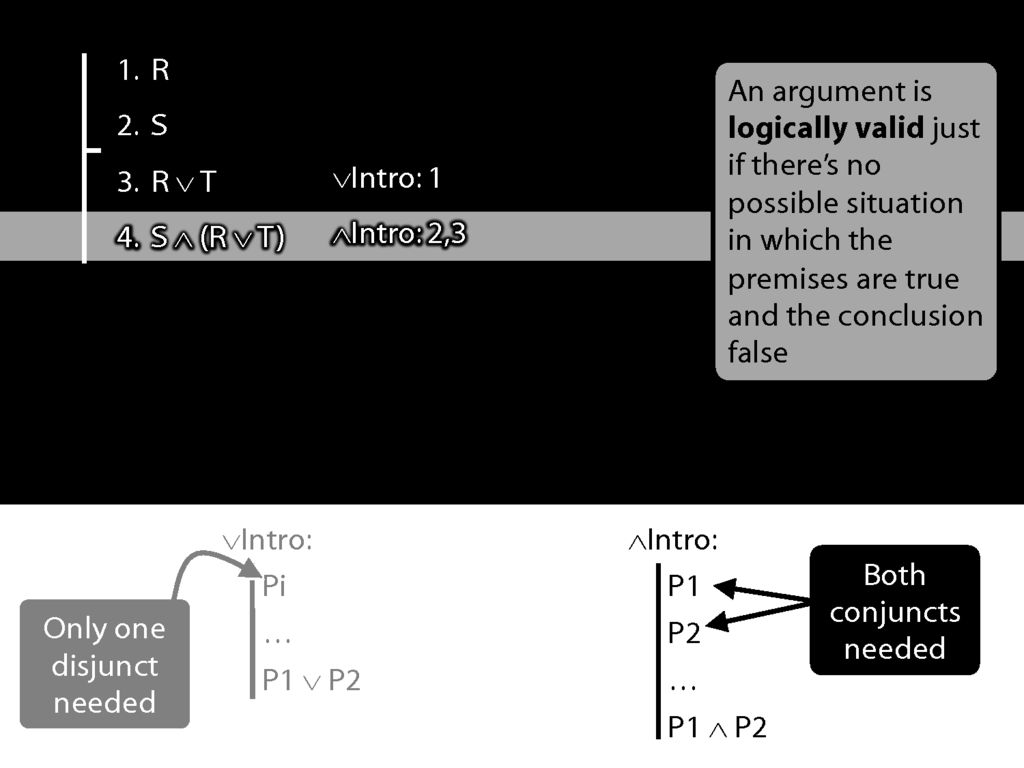Now we're using ∧Intro.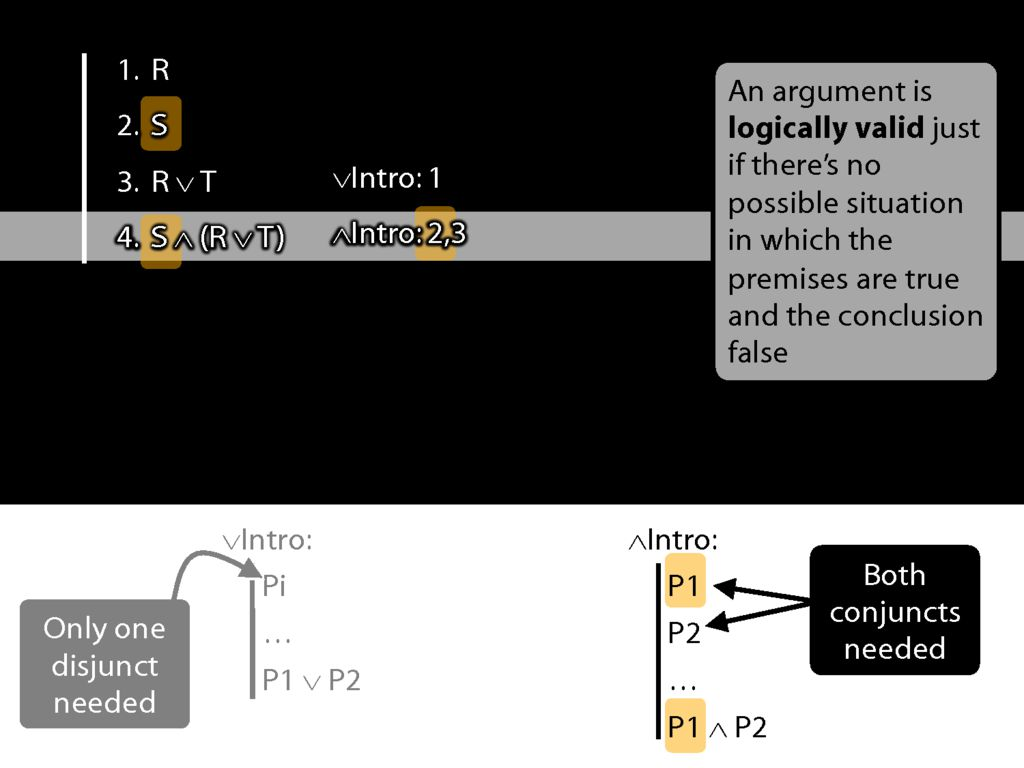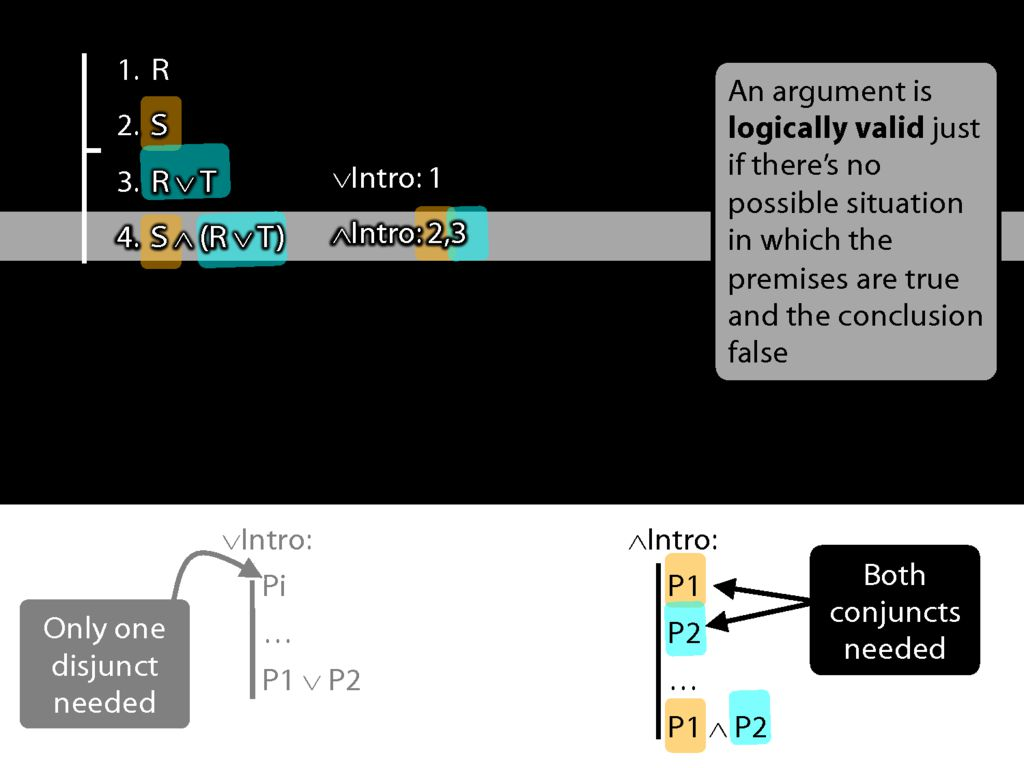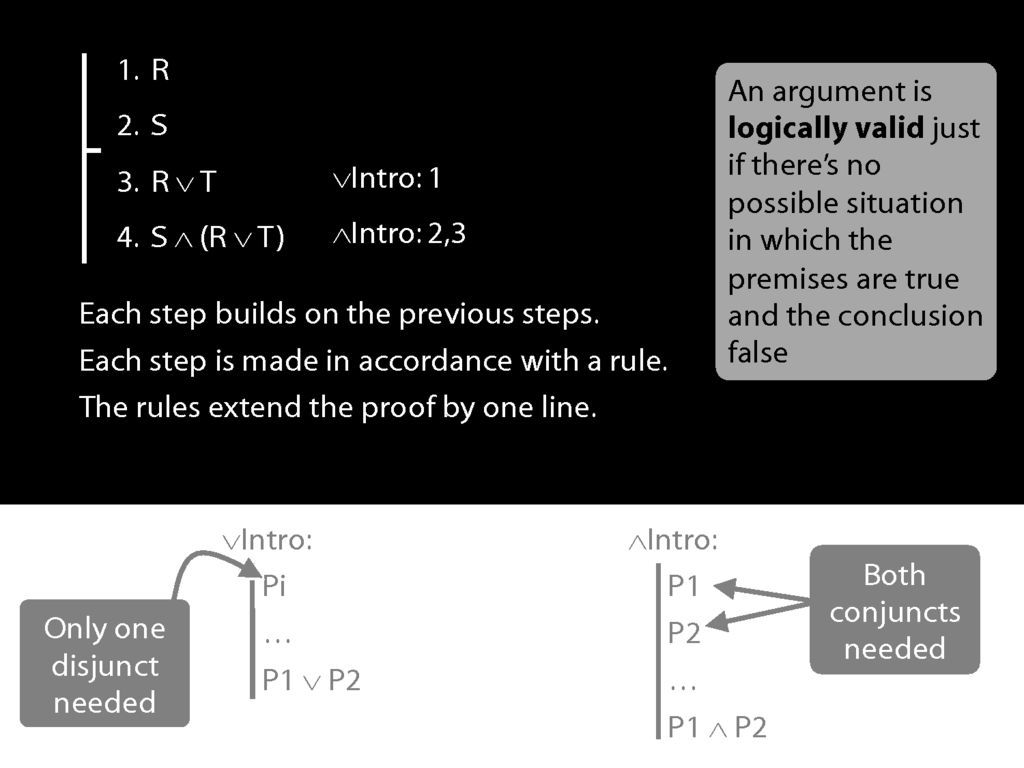Note some properties of the proof:
Each step builds on the previous steps; each step is made in accordance with a rule; and the rules we've seen so far extend the proof by one line.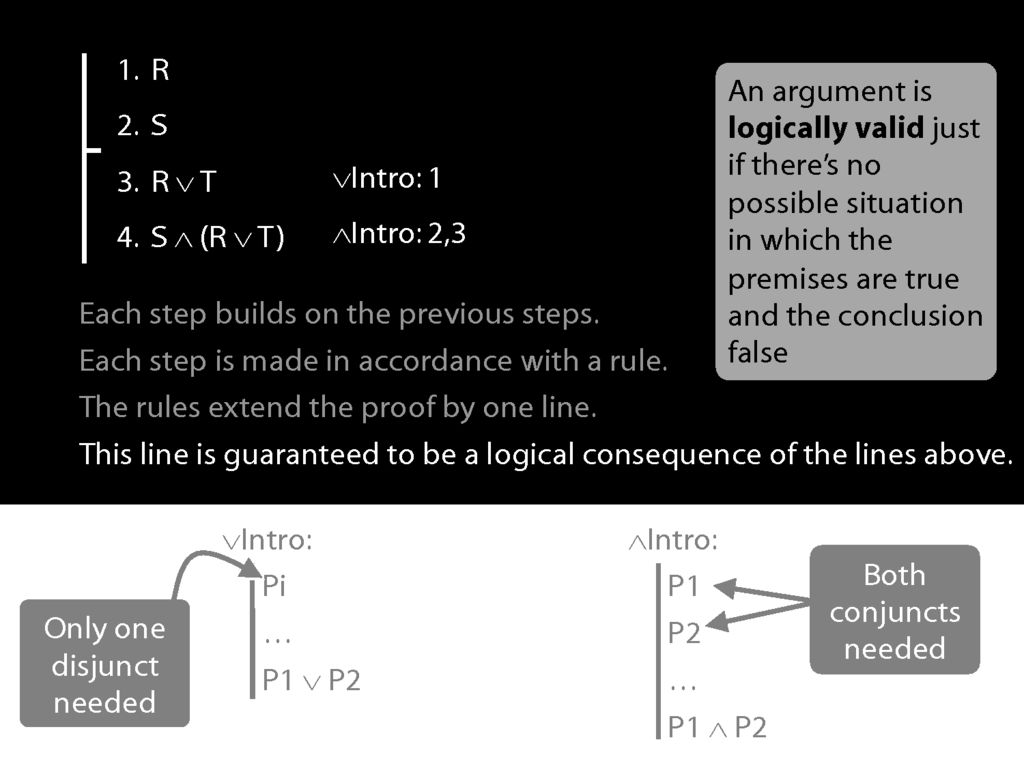The most important property, though is this: The new line is guaranteed to be a logical consequence of the lines above.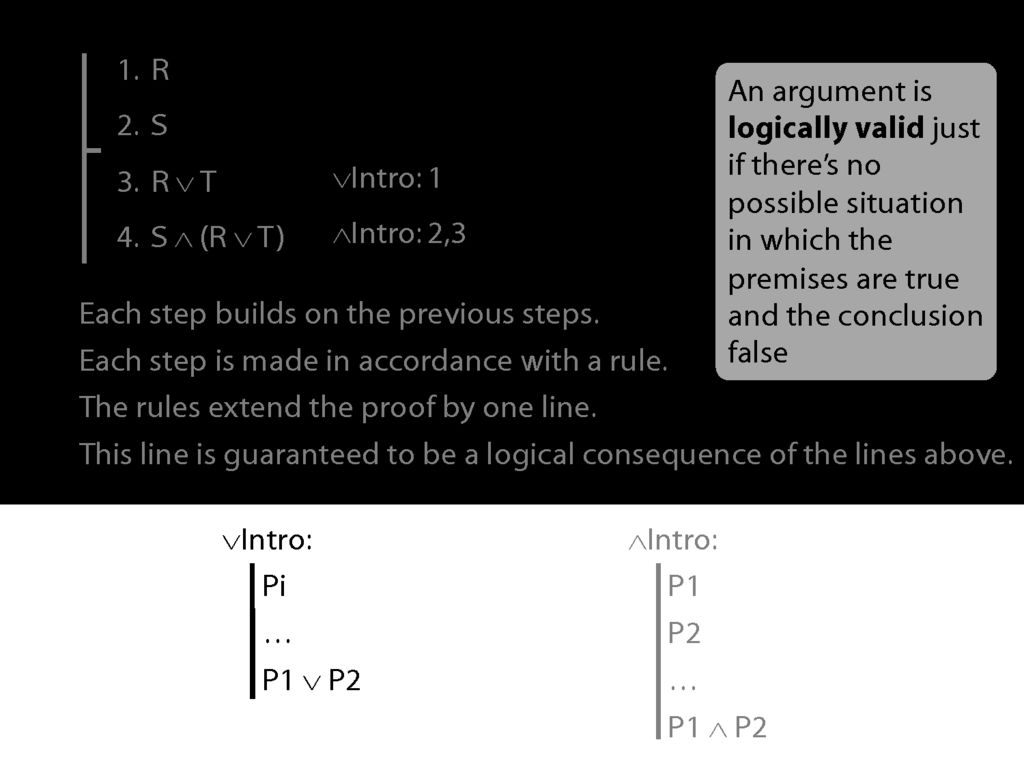Let me illustrate ...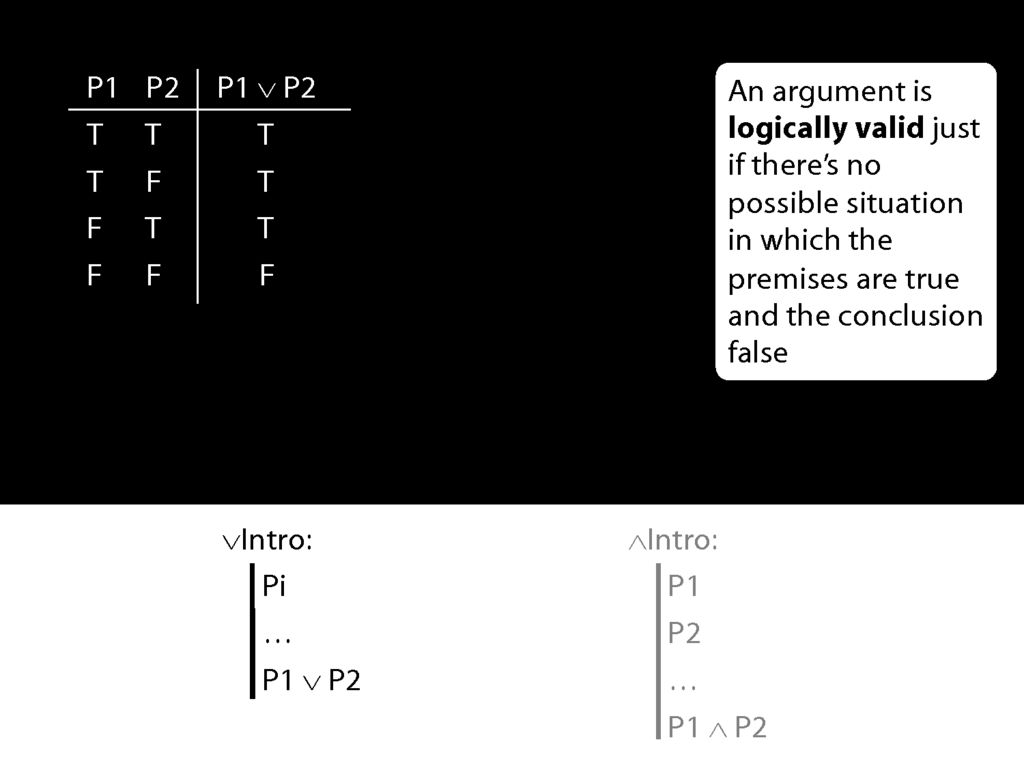Consider the truth table for disjunction.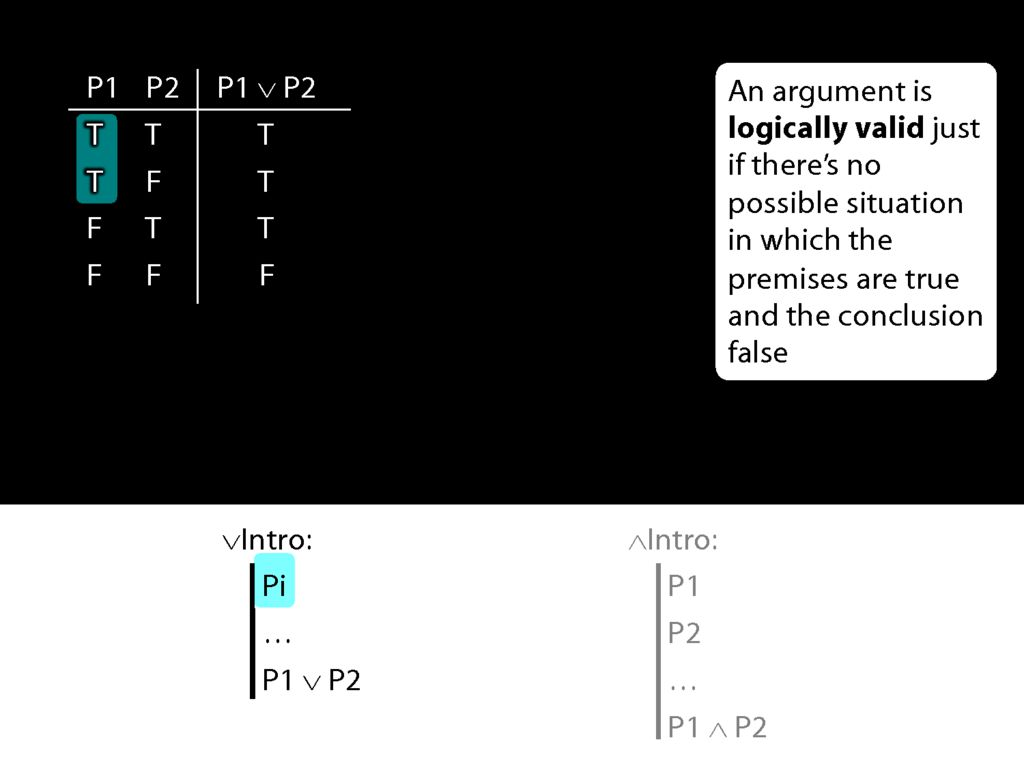What happens when the prerequiste for the disjunction-intro rule is true? In that case, we're in the first two rows of the truth table.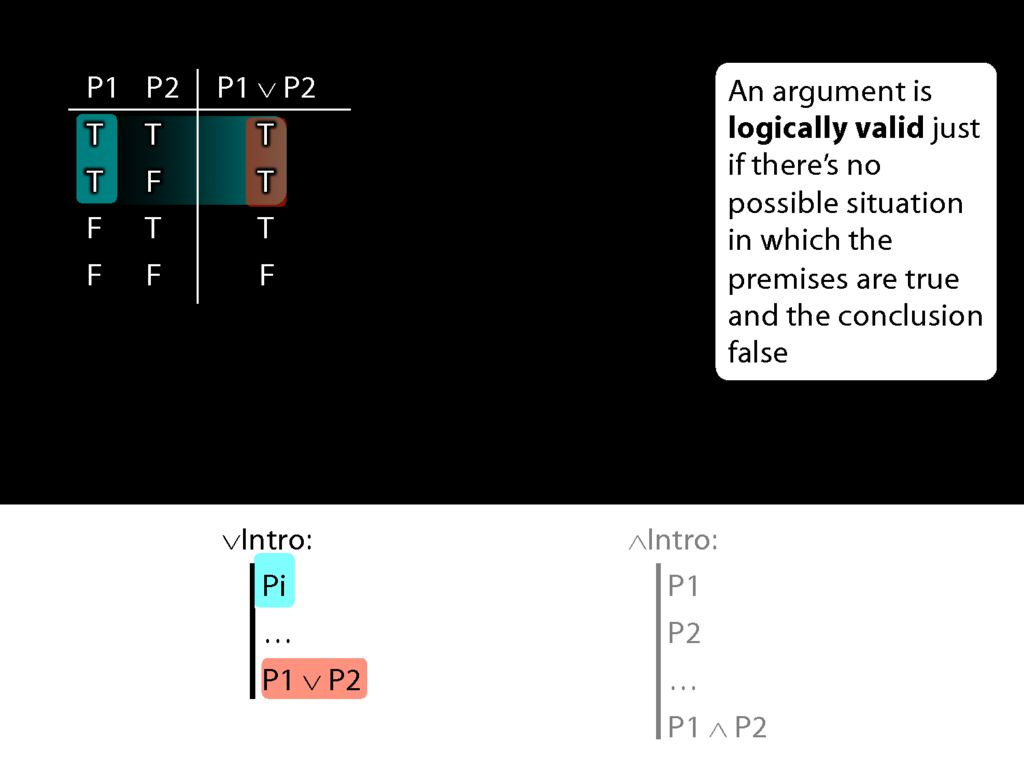And in those first two rows, the disjunction which the rule adds to the proof is true.
So given the truth table, we can see that disjunction-intro will never take us from a truth to a falsehood; from a truth we can only reach another truth using disjunction-intro.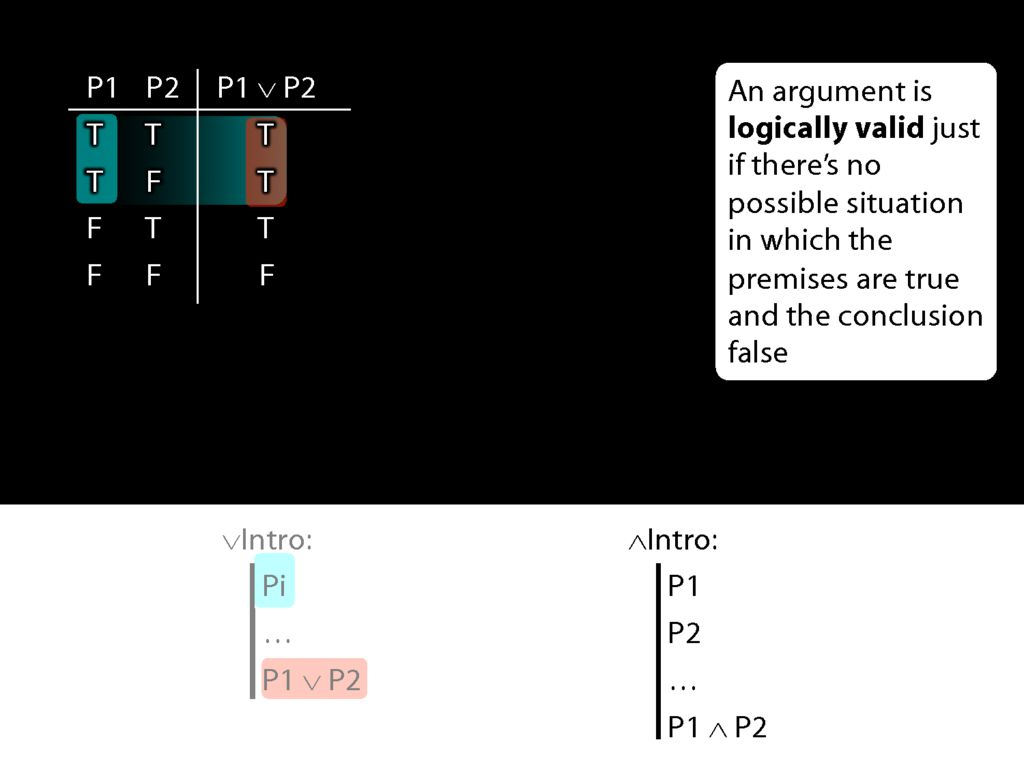Now look at conjunction intro.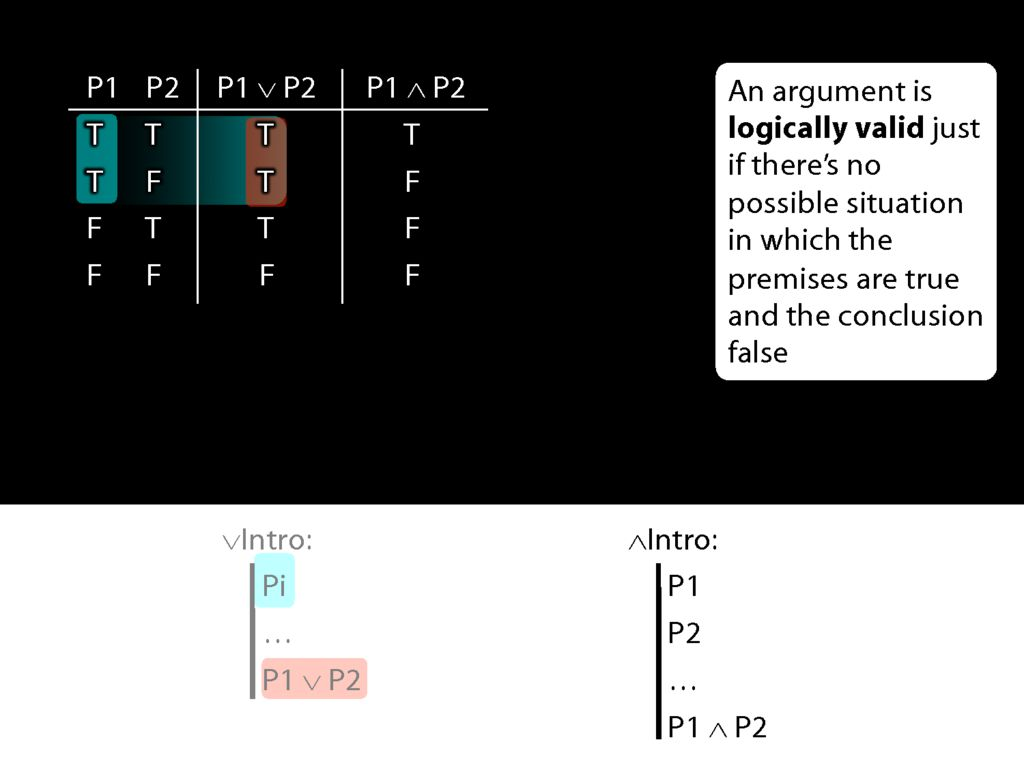Here's the truth table for conjunction.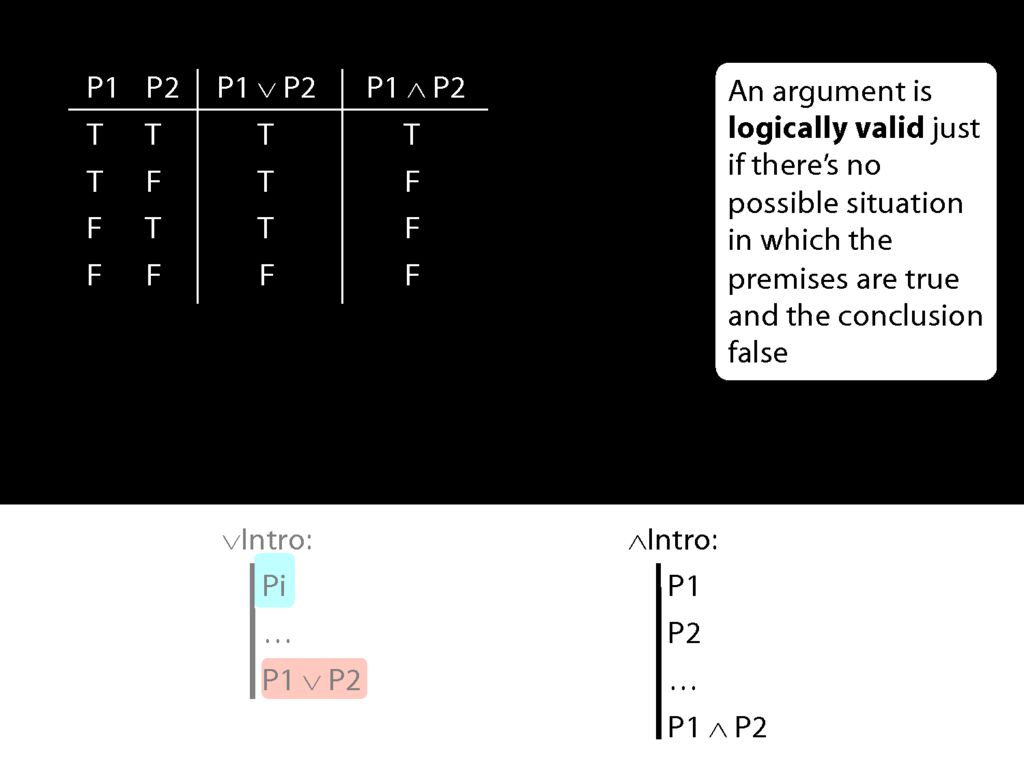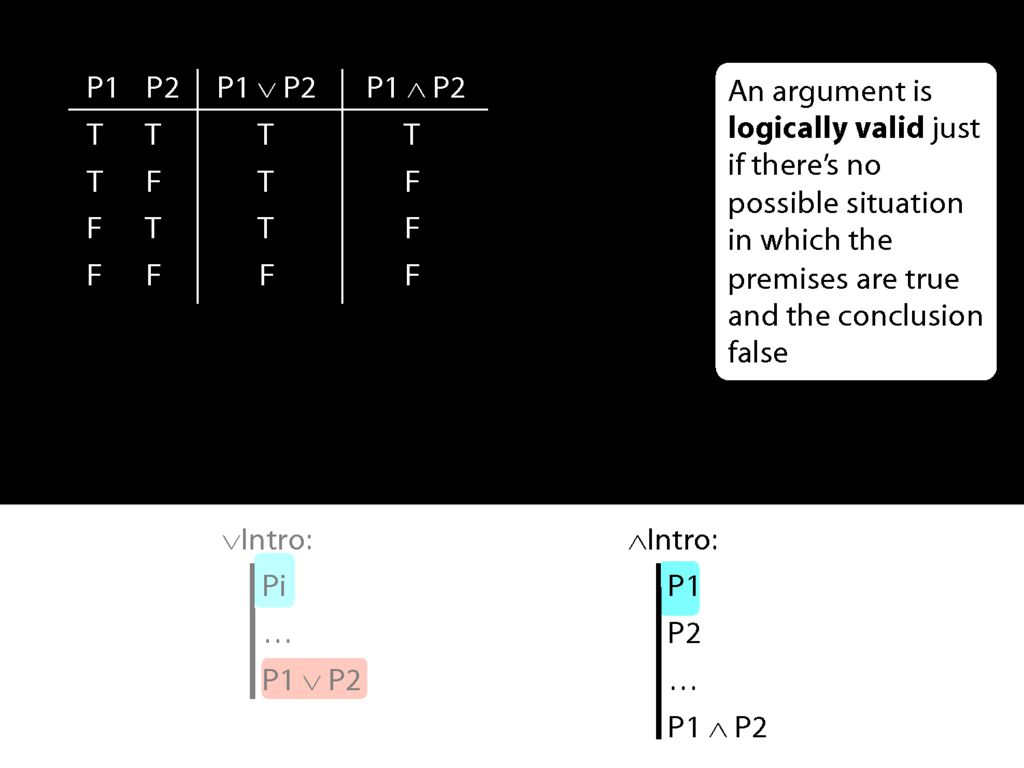Now what happens when the first prerequisite for conjunction introduction is true?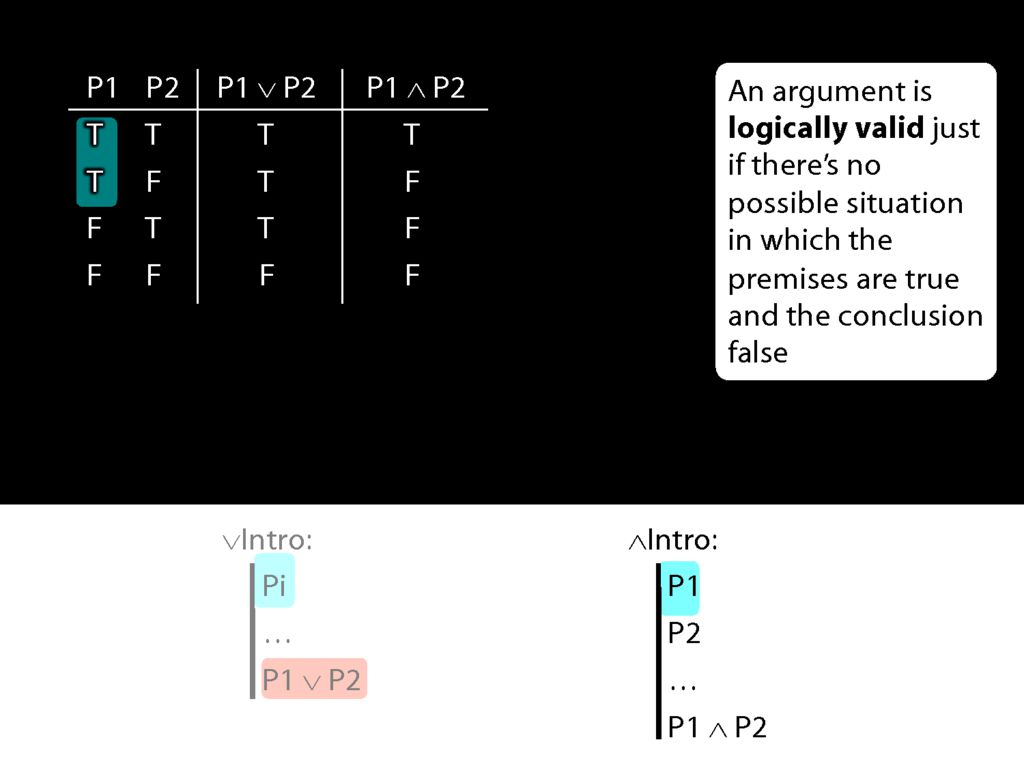In that case, we're in the first two rows of the truth table.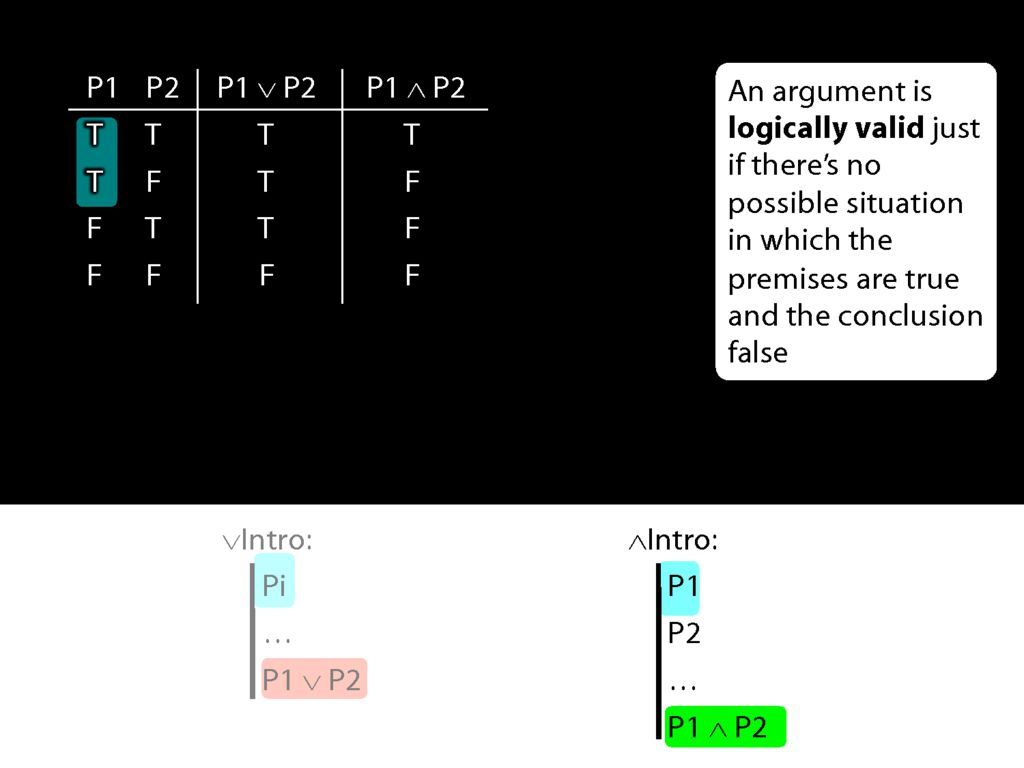What is the truth value of the conjunction which conjunction-introduction adds to the proof in these rows of the truth table?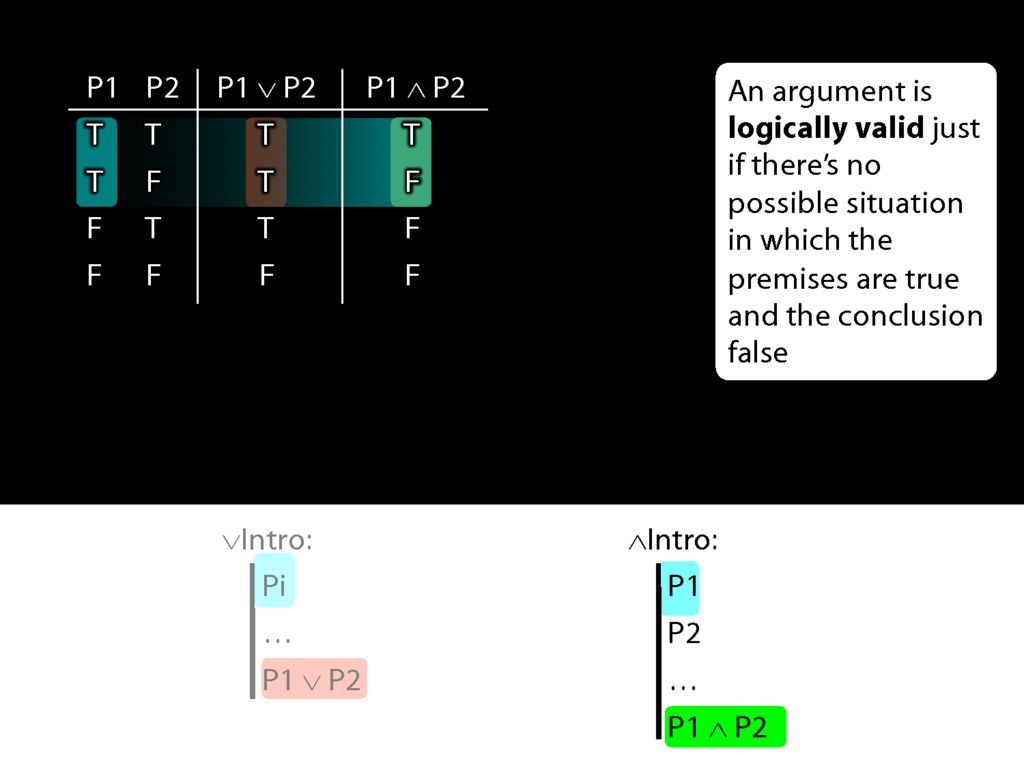Actually this conjunction is true is one row and false in the other.
So when P1 is true, P1∧P2 is sometimes true and sometimes false.
This means that if conjunction-intro required just one conjunct, it would sometimes take us from a truth to a falsehood.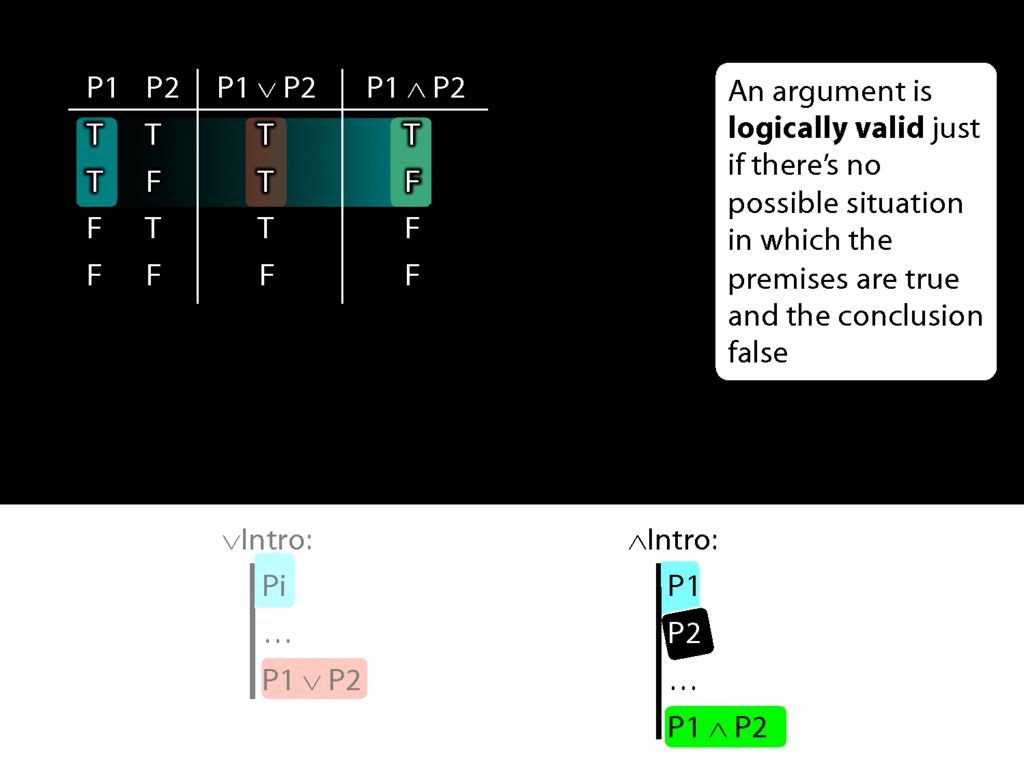But actually conjunction-intro requires that we already have both conjuncts in our proof.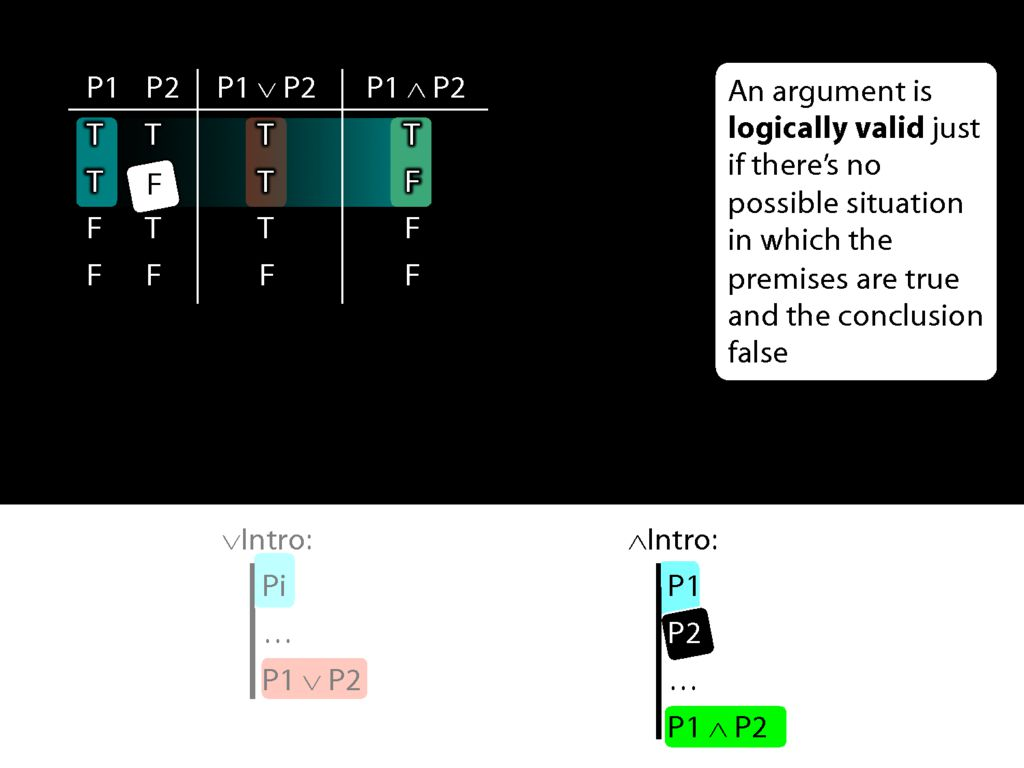So if both pre-requisites for using conjuncton introduction are true, then we can't be in the second row of the truth table.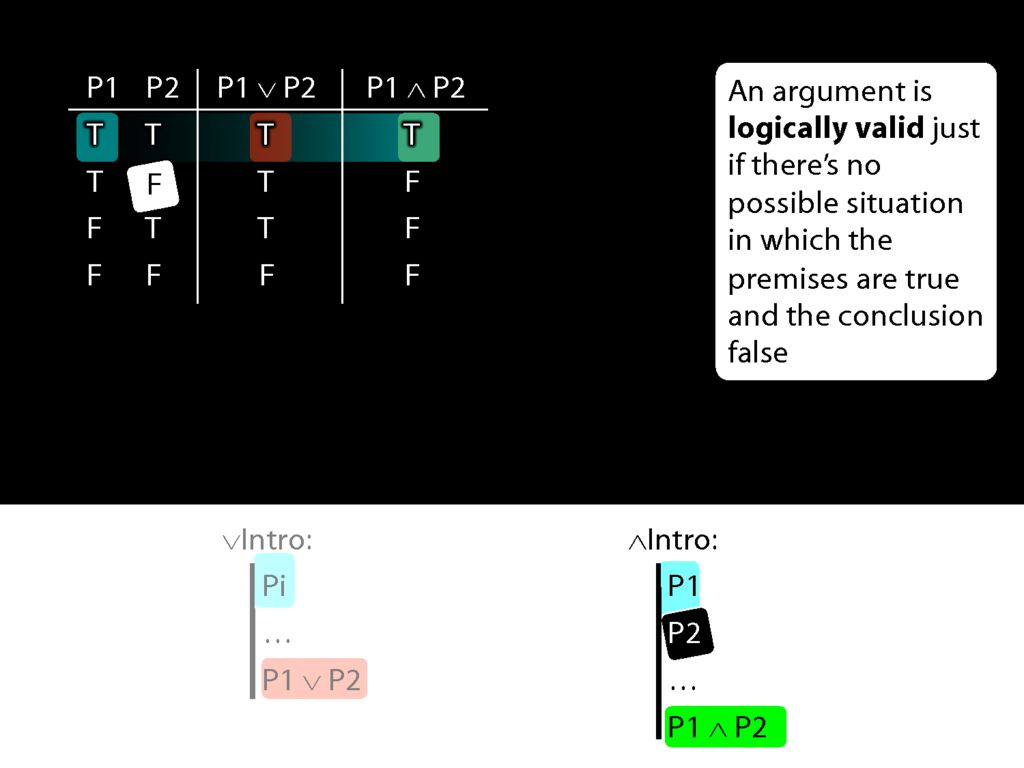And this means that when everything conjunction-intro requires is true, then the conjunction it allows us to introduce must always be true.
Earlier I asked, Why is using ∧Intro more demanding than using ∨Intro? Now we can see exactly what the answer is.
Speaking entirely formally, we can use whatever rules we like.
But we want our rules to mirror logical validity--given truths, we want them to take us only to truths. We want them never to take us from truth to falsehood.
For this reason, given the truth table for conjunction, ∧Intro has to require that we already have both conjuncts.Let me make the same point by introducing a new connective.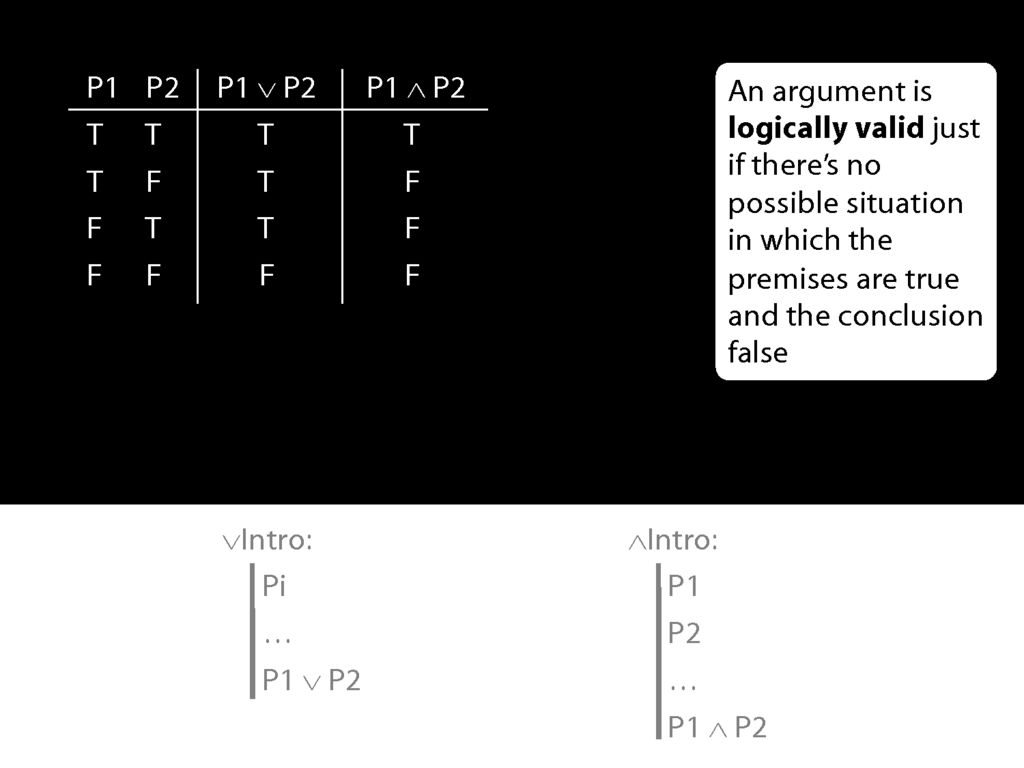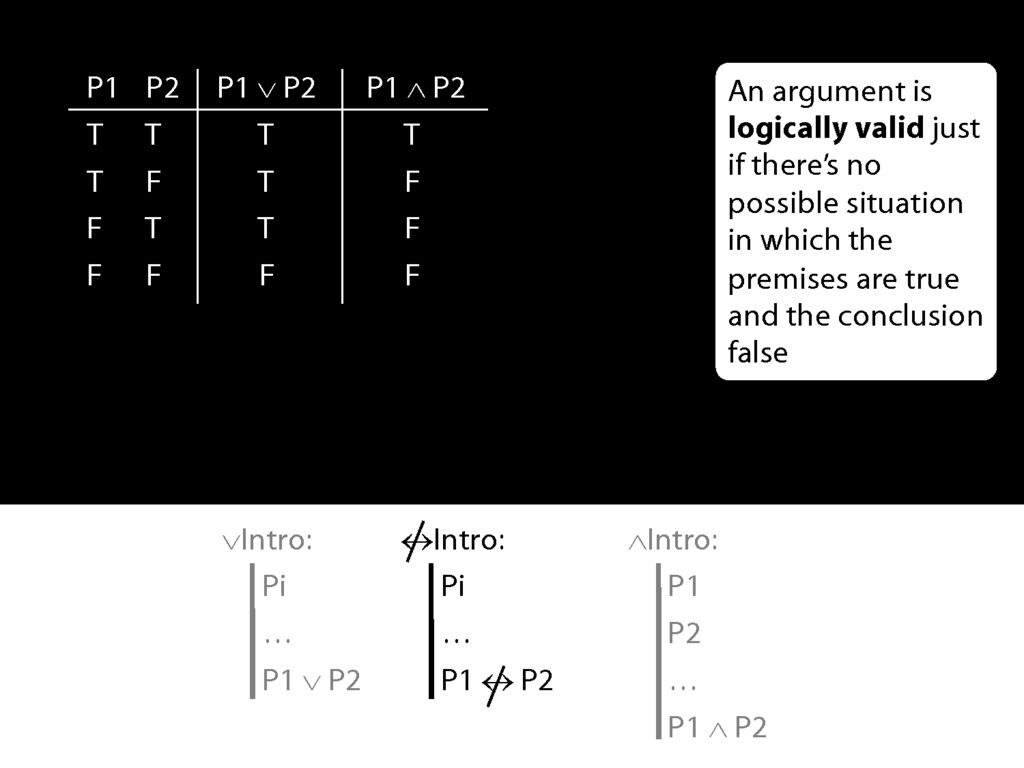Suppose we have a connective called 'is not eqivalent' and we stipulate this introduction rule.
\begin{minipage}{\columnwidth}
Let us define a new connective with this truth table:
\end{minipage}
\begin{minipage}{\columnwidth}
The following rule is unacceptable. Why?
\end{minipage}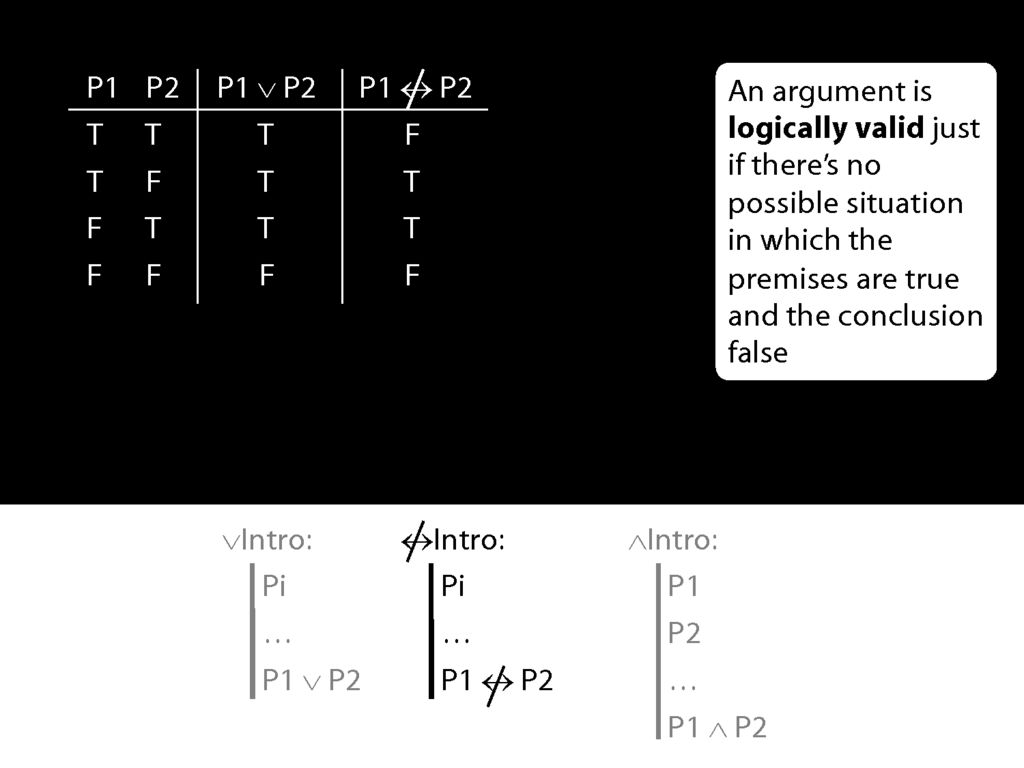Suppose also that our new connective has this truth table, so that the 'P1 is not eqivalent to P2' is true exactly when P1 and P2 have different truth values.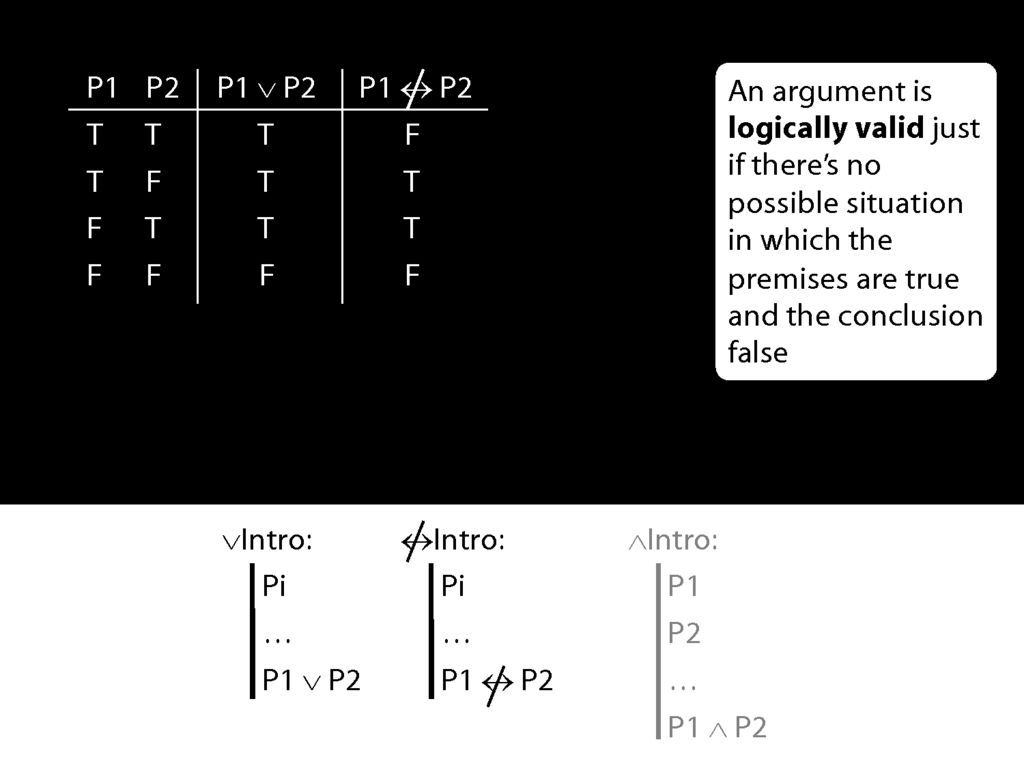Now the rule for not-equivalent-intro is just like the rule for disjunction-intro.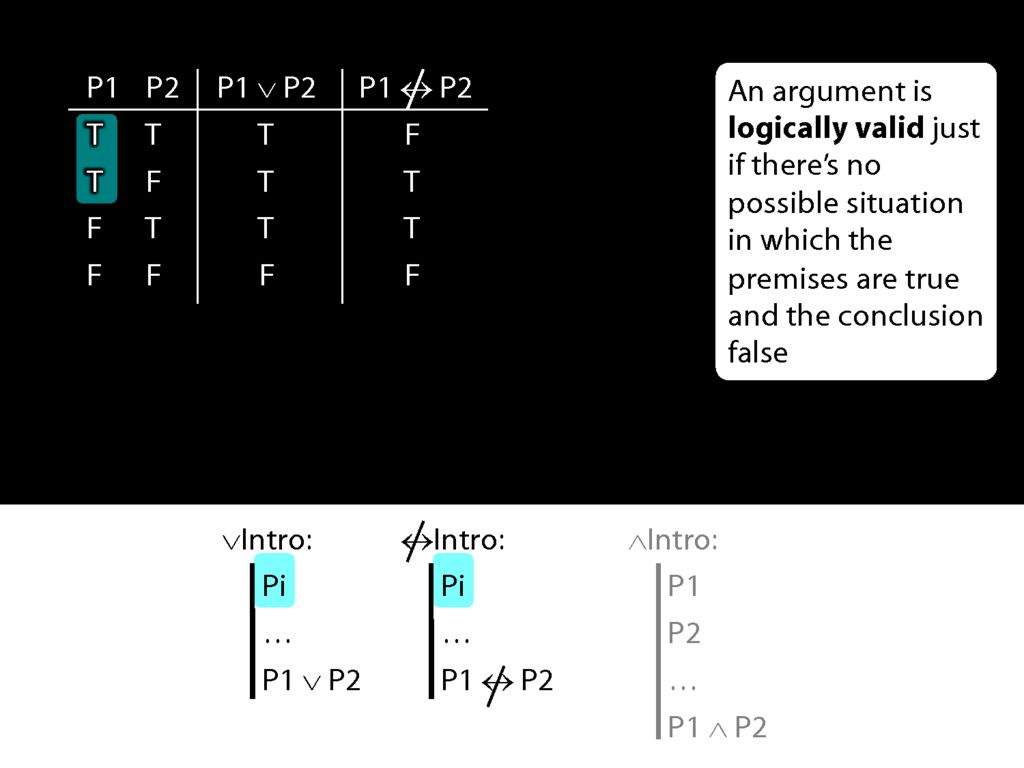What happens when the prerequsite for these rules is true?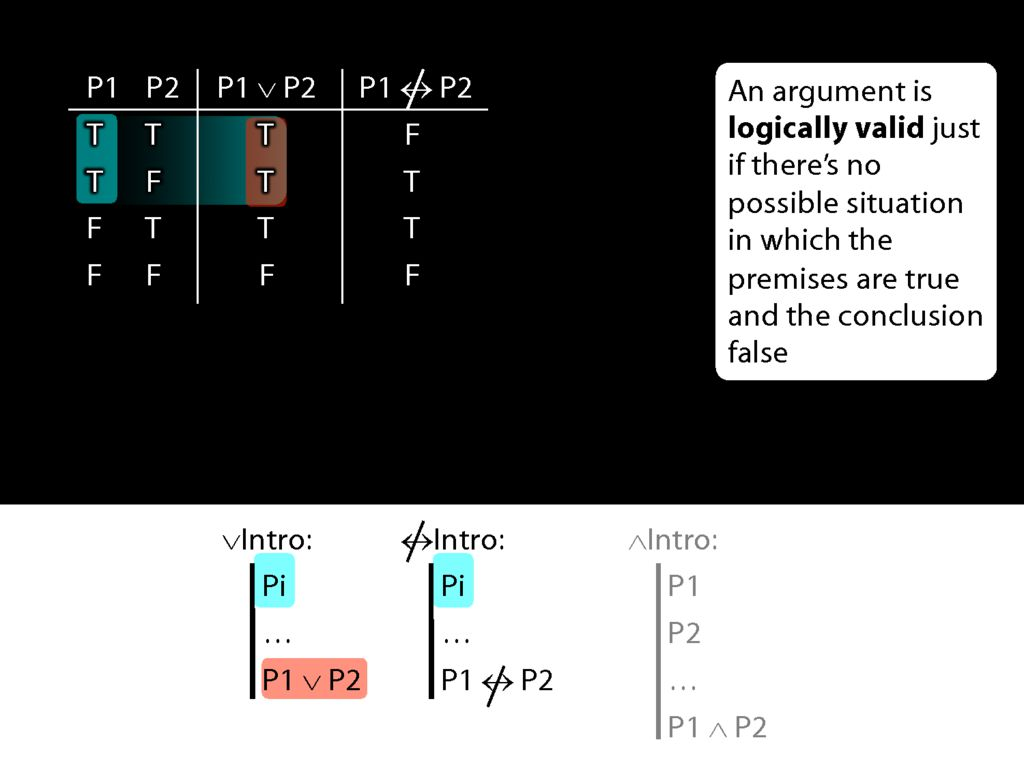The disjunction that we can introduce with disjunction-intro is true.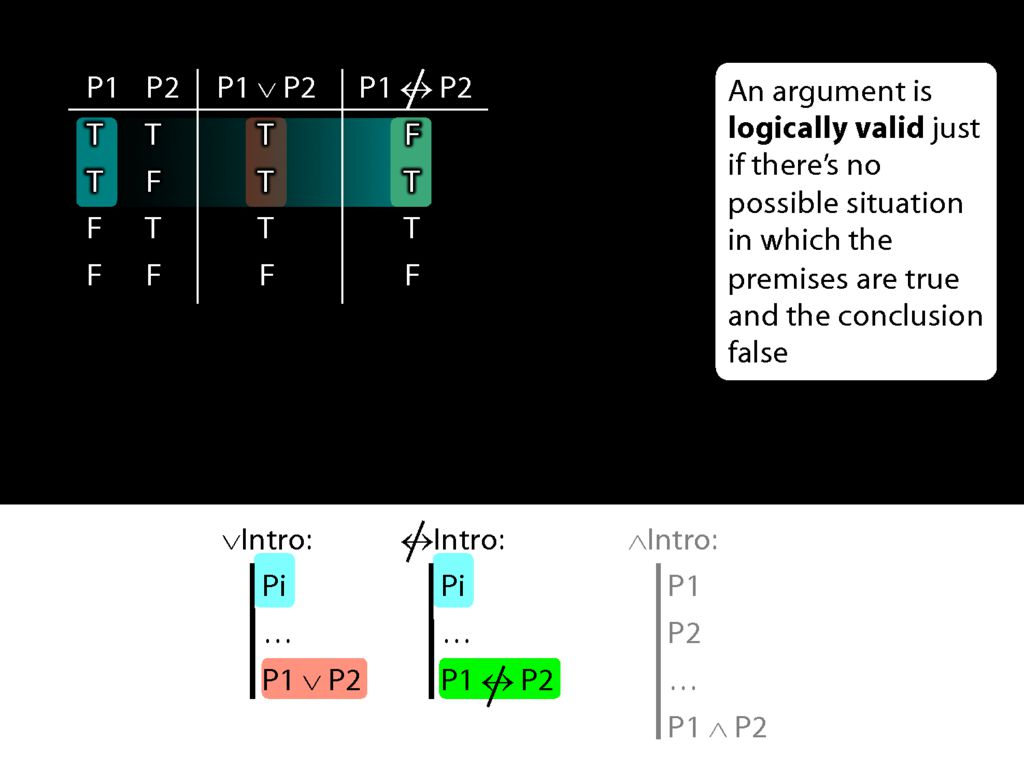But the sentence that we can introduce with not-equivalent-intro is sometimes false and sometimes true.
So the new rule not-equivalent-intro would allow us to move from truths to falsehoods.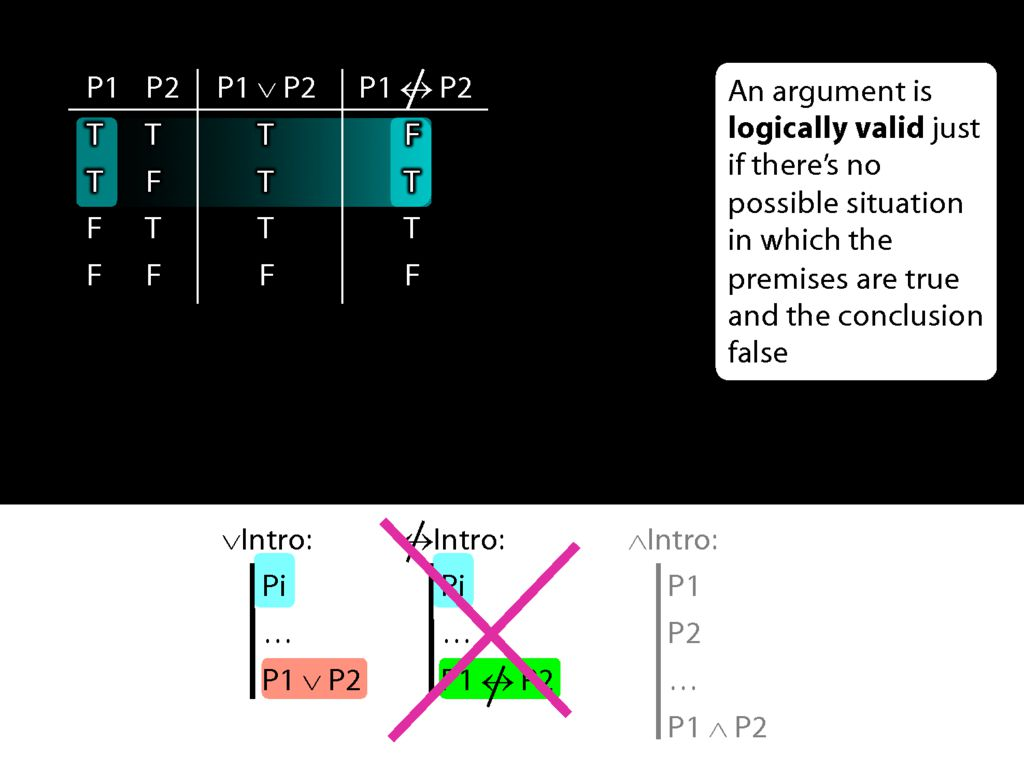That's why we shouldn't have this rule.